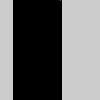Name

### noise()

Examples```xoff = 0.0
background(204)
for i in range(1000):
xoff = xoff + .01
n = noise(xoff) * width
line(n, 0, n, height)
```
```noiseScale = 0.02
def draw():
background(0)
for x in range(width):
noiseVal = noise((mouseX + x) * noiseScale, mouseY * noiseScale)
stroke(noiseVal * 255)
line(x, mouseY + noiseVal * 80, x, height)
```
Description Returns the Perlin noise value at specified coordinates. Perlin noise is a random sequence generator producing a more natural, harmonic succession of numbers than that of the standard random() function. It was invented by Ken Perlin in the 1980s and has been used in graphical applications to generate procedural textures, shapes, terrains, and other seemingly organic forms.

In contrast to the random() function, Perlin noise is defined in an infinite n-dimensional space, in which each pair of coordinates corresponds to a fixed semi-random value (fixed only for the lifespan of the program). The resulting value will always be between 0.0 and 1.0. Processing can compute 1D, 2D and 3D noise, depending on the number of coordinates given. The noise value can be animated by moving through the noise space, as demonstrated in the first example above. The 2nd and 3rd dimensions can also be interpreted as time.

The actual noise structure is similar to that of an audio signal, in respect to the function's use of frequencies. Similar to the concept of harmonics in physics, Perlin noise is computed over several octaves which are added together for the final result.

Another way to adjust the character of the resulting sequence is the scale of the input coordinates. As the function works within an infinite space, the value of the coordinates doesn't matter as such; only the distance between successive coordinates is important (such as when using noise() within a loop). As a general rule, the smaller the difference between coordinates, the smoother the resulting noise sequence. Steps of 0.005-0.03 work best for most applications, but this will differ depending on use.
Syntax
```noise(x)
noise(x, y)
noise(x, y, z)
```
Parameters
x float: x-coordinate in noise space float: y-coordinate in noise space float: z-coordinate in noise space
Related noiseSeed()
noiseDetail()
random()

Updated on Mon Sep 21 15:53:25 2020.

If you see any errors or have comments, please let us know.